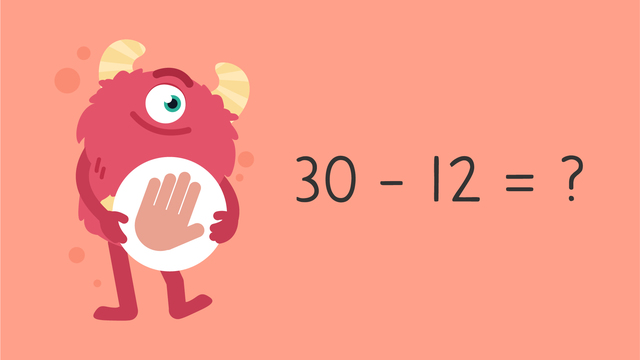# Make a Number Line to Subtract — Let's Practice!Rating

Ø 5.0 / 1 ratings
The authorsTeam Digital

### TranscriptMake a Number Line to Subtract — Let's Practice!

Razzi says get these items ready (...) Because today we're going to practice... : "Make a number line to subtract". It's time to begin! There are many strategies and tools to help subtract, like using a number line. Remember, when subtracting using a number line, ALWAYS start by putting the larger number on the RIGHT. Then, count back small amounts of the smaller number. If Mr. Squeaks has thirty seeds, but only need twelve, how many will he have left over? Did you also get eighteen? Let's tackle the next problem! Mr. Squeaks has forty-six sticks but only needs twenty, how many will he have left? Remember, when subtracting using a number line, ALWAYS start by putting the larger number on the RIGHT. Then, count back small amounts of the smaller number. Did you also get twenty-six? Let's tackle the last problem! If Mr. Squeaks has sixty nails but only needs twenty-eight, how many will he have left? Remember, when subtracting using a number line, ALWAYS start by putting the larger number on the RIGHT. Then, count back small amounts of the smaller number. Did you also get thirty-two? Razzi had so much fun practicing with you today! See you next time!

## Make a Number Line to Subtract — Let's Practice! exercise

Would you like to apply the knowledge you’ve learned? You can review and practice it with the tasks for the video Make a Number Line to Subtract — Let's Practice!.
• ### Razzi subtracts.

Hints

Start by subtracting 10 from 26, what do you get?

Then, subtract 10 again. What is the answer?

Solution

26 - 20 = 6

• Start by putting the larger number, 26 on the right.
• Count back small amounts of the smaller number, 20.
• 20 can be broken up into two groups of 10.
• 26 - 20 = 6
26 - 10 = 16, 16 - 10 = 6 therefore 26 - 20 = 6.

• ### Subtract on the number line.

Hints

Count back small amounts of the smaller number, 14.

14 can be broken up into a group of 10 and 4 ones.

Solution

40 - 14 = 26

• Start by putting the larger number, 40 on the right.
• Count back small amounts of the smaller number, 14.
• 14 can be broken up into a group of 10 and 4 ones.
• 40 - 10 = 30
• 30 - 4 = 26

• ### Help Mr. Squeaks subtract seeds.

Hints

Count back small amounts of the smaller number, 36.

36 can be broken up into 30, 5, and 1.

Subtract 30 from 80, what do you get? Fill this in and continue subtracting.

Solution

80 - 36 = 44

• Start by putting the larger number, 80 on the right.
• Count back small amounts of the smaller number, 36.
• 36 can be broken up into 30 and 6.
• 80 - 30 = 50
• 50 - 5 = 45
• 45 - 1 = 44

• ### Mr. Squeak's stickers.

Hints

What should you subtract from 100?

Count back small amounts of the smaller number, 29.

29 can be broken up into 20, 5 and 4 ones.

Solution

100 - 29 = 71

• Start by putting the larger number, 100 on the right.
• Count back small amounts of the smaller number, 29.
• 29 can be broken up into 20 and 9.
• 100 - 20 = 80
• 80 - 5 = 75
• 75 - 4 = 71

• ### Steps for subtracting on the number line.

Hints

Should you start with the larger number, 43 or the smaller number, 16 on the number line?

Before you count back, what do you need to do?

Solution

Here are the steps for subtracting using a number line:

• Start by putting the larger number, 43 on the right.
• Break 16 into one group of ten and 6 ones.
• Count back small amounts of the smaller number, 16.
• 43 - 10 = 33
• 33 - 6 = 27
• ### Solve the two part problem.

Hints

27 can be broken up into 20 and 7.

• Solve for 40 - 27.
• Subtract 3 from the total.
Solution

40 - 27 - 3 = 10

• Start by putting the larger number, 40 on the right.
• Count back small amounts of the smaller number, 27.
• 27 can be broken up into 20 and 7.
• 40 - ? = 20
• 40 - 20 = 20
• 20 - 7 = 13
• 13 - 3 = 10